# RD Sharma Solutions For Class 12 Maths Exercise 19.17 Chapter 19 Indefinite Integrals

RD Sharma Solutions for Class 12 Maths Exercise 19.17 Chapter 19 Indefinite Integrals is provided here. Students who wish to develop a strong grip over the concepts of Maths can use RD Sharma Solutions for this purpose. This resource can also be used by students for preparation of their exams.

All the RD Sharma Solutions are prepared by subject experts at BYJU’S to match all levels of students. This exercise deals with examining the integrals of the type

$$\begin{array}{l}\int \frac{1}{\sqrt{ax^{2} + bx + c}} \ dx\end{array}$$
with algorithm. The PDF of RD Sharma Solutions for Class 12 Maths Chapter 19 Exercise 19.17 is available here.

## Download the PDF of RD Sharma Solutions For Class 12 Chapter 19 – Indefinite Integrals Exercise 19.17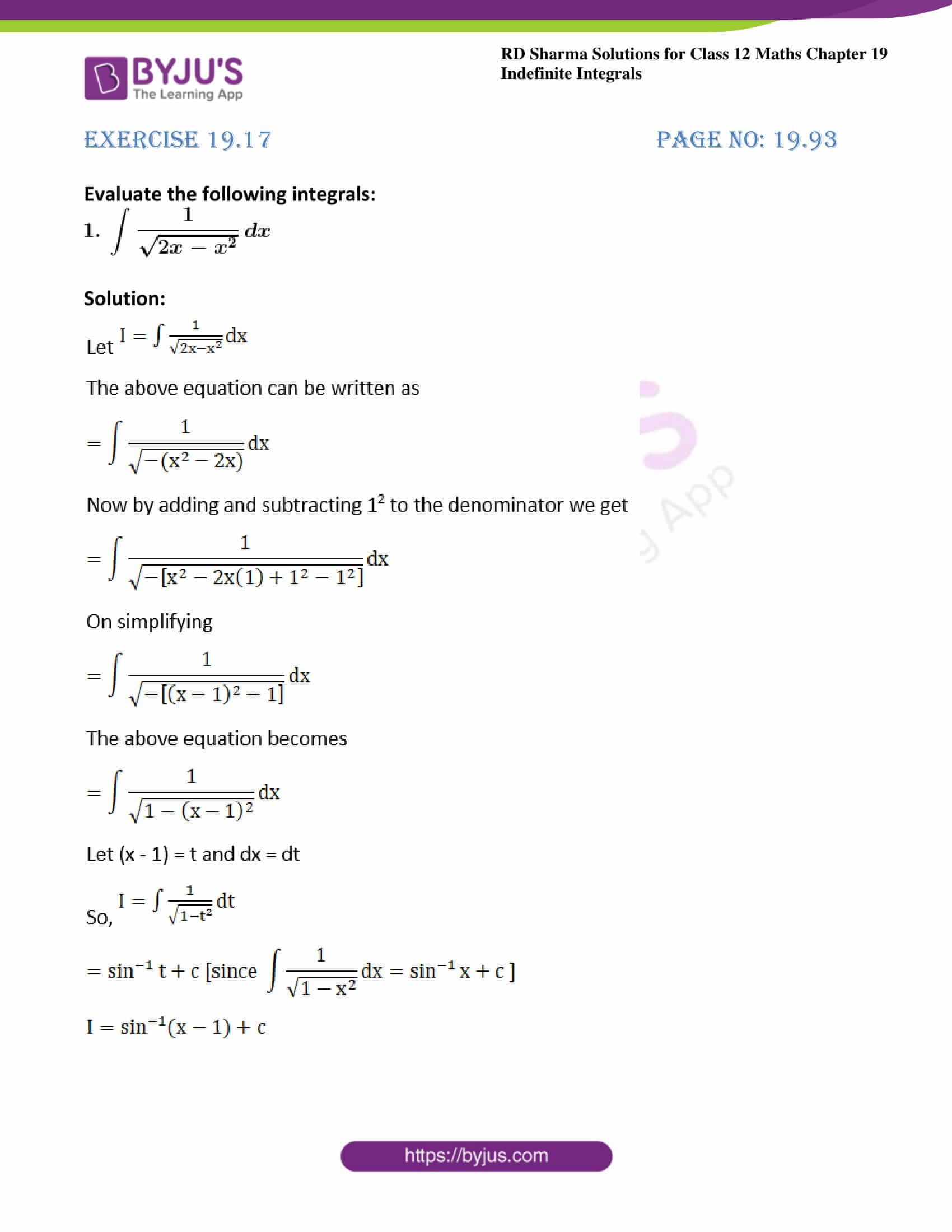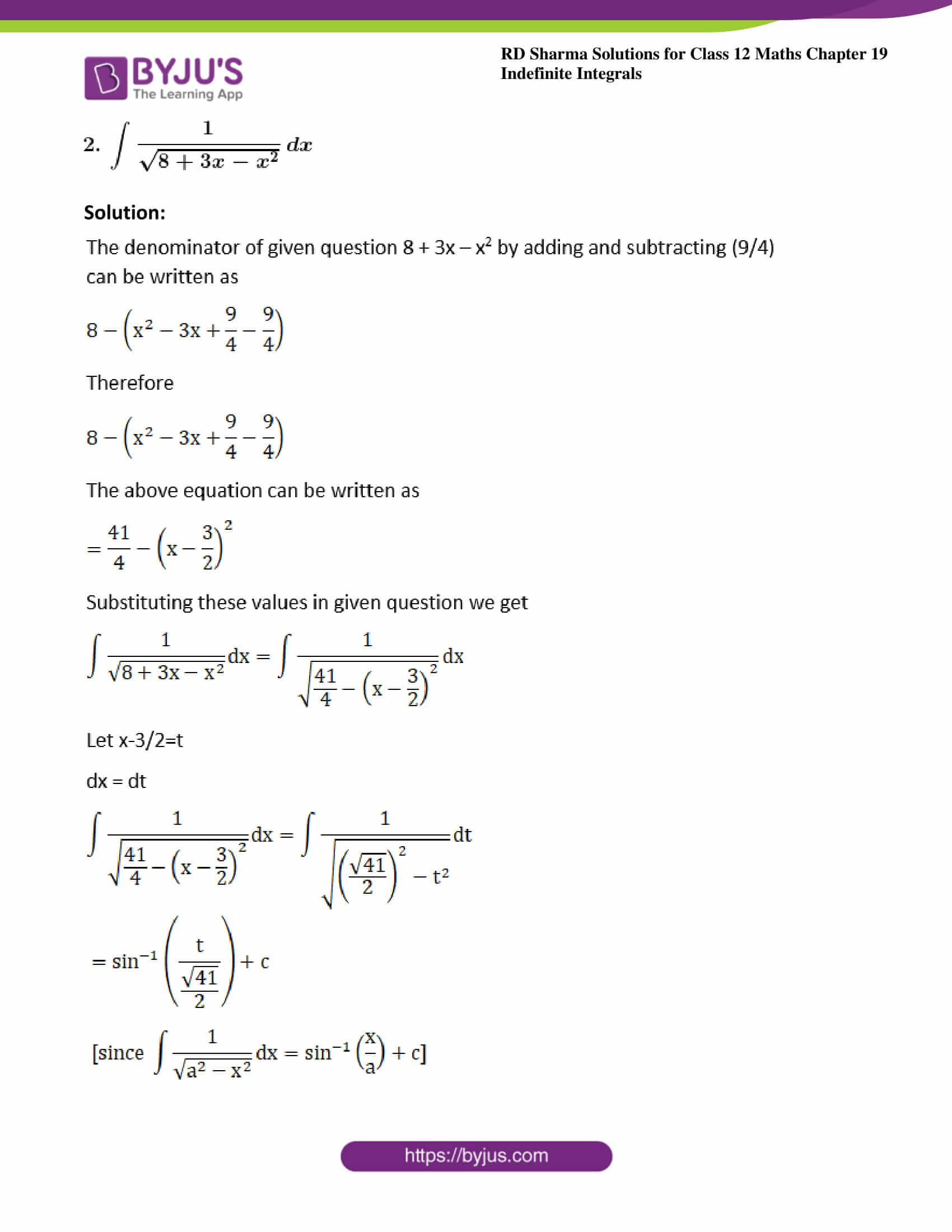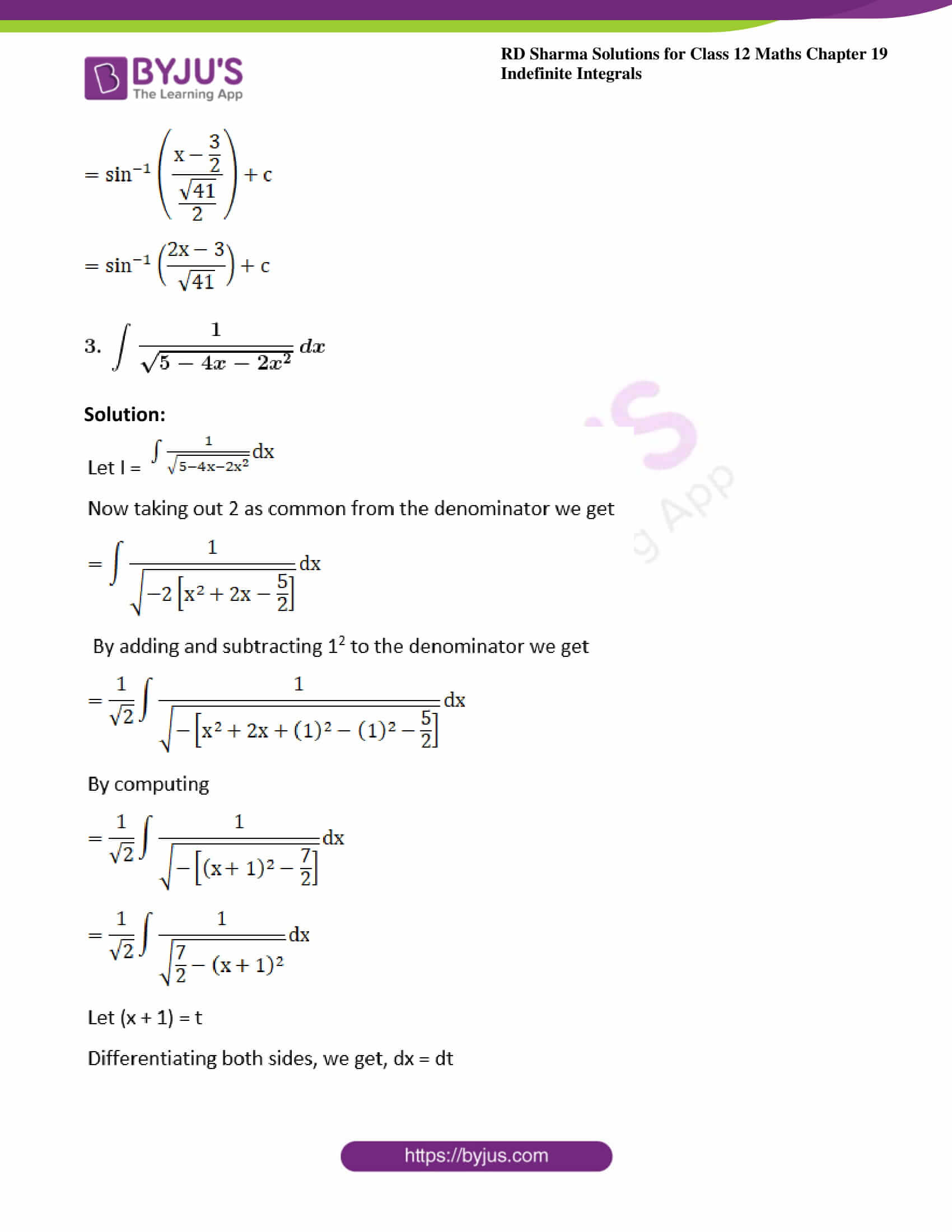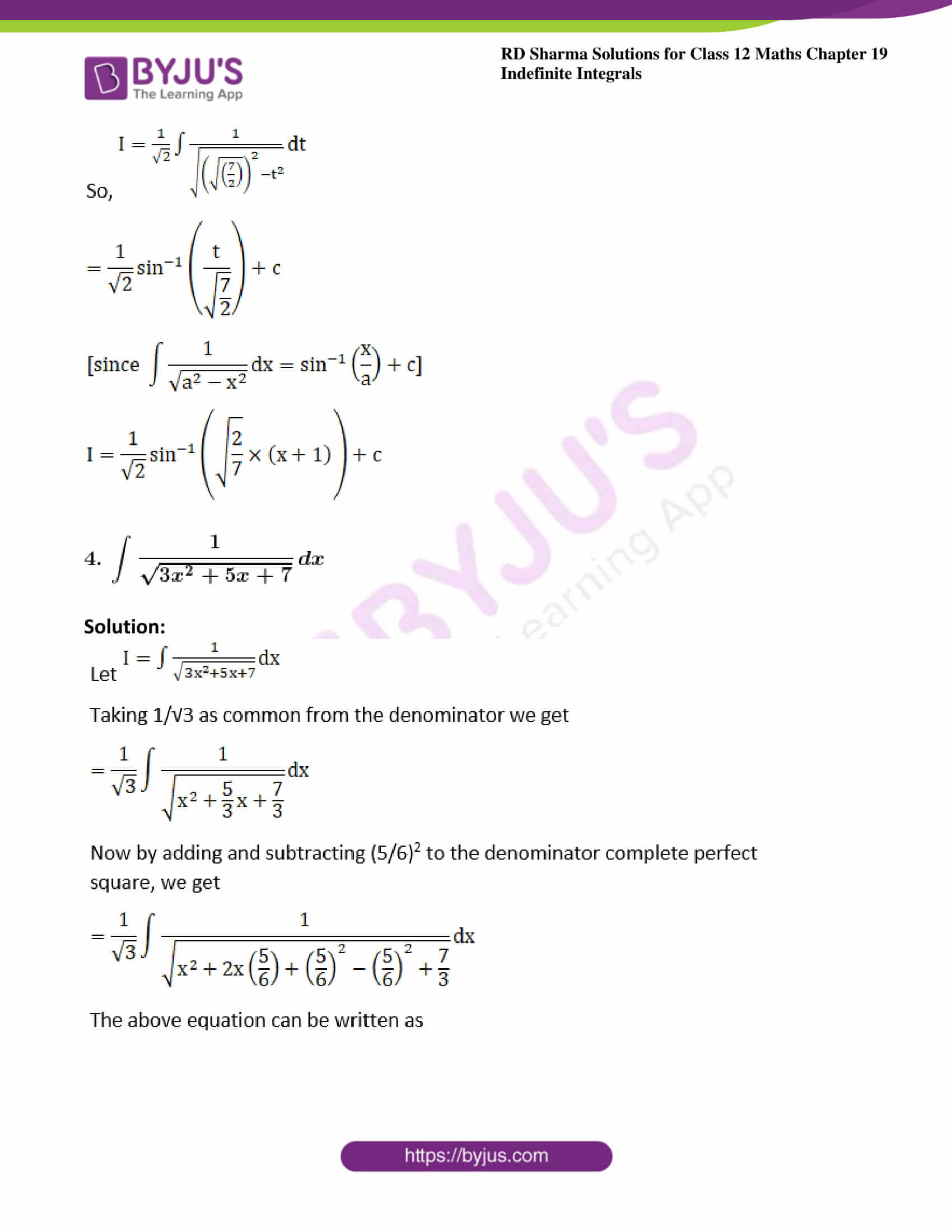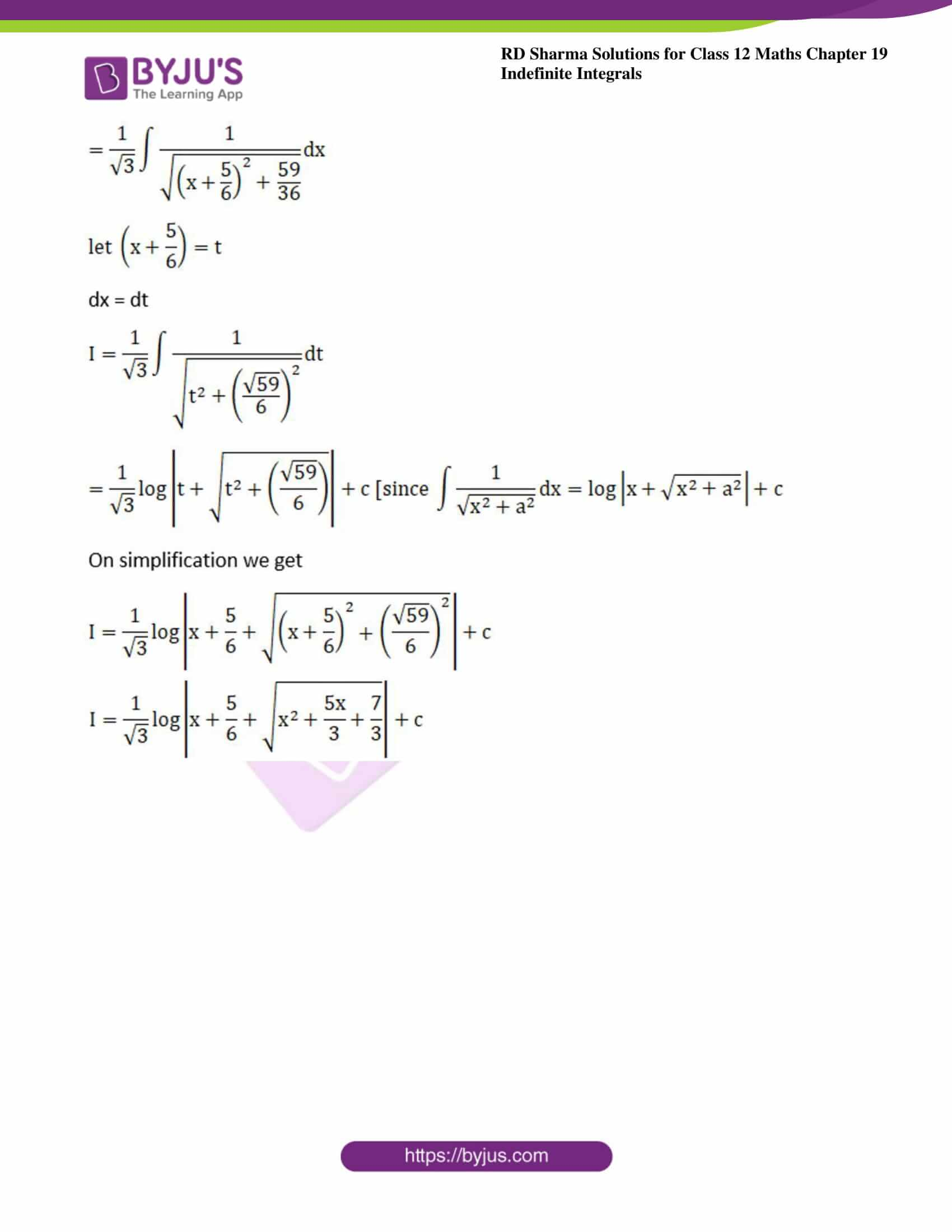### Exercise 19.17 Page No: 19.93

Evaluate the following integrals: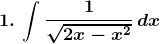Solution: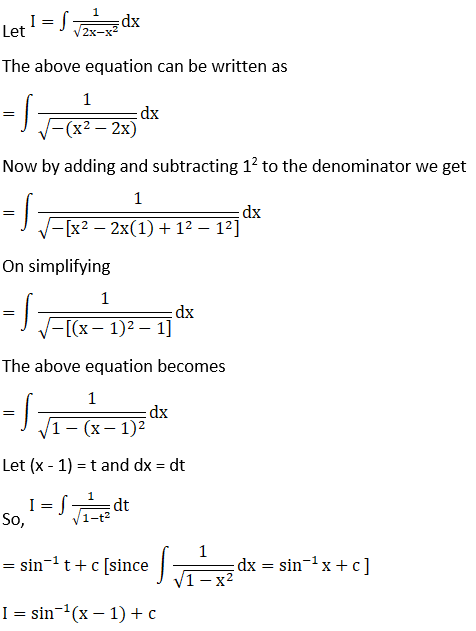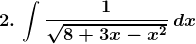Solution: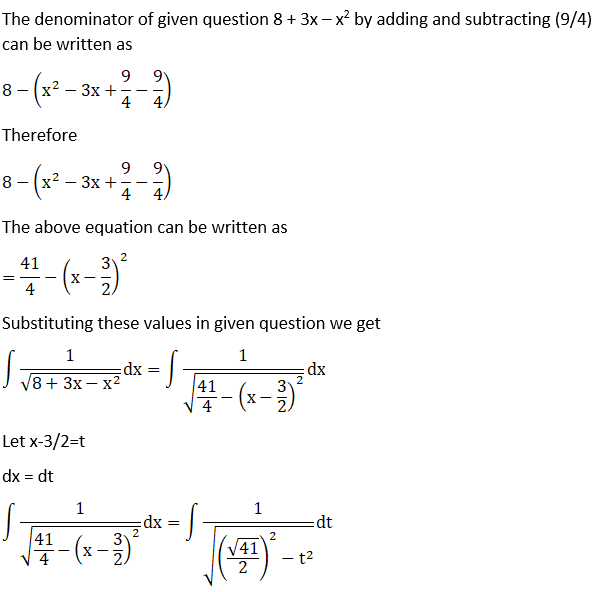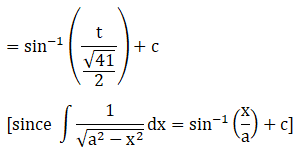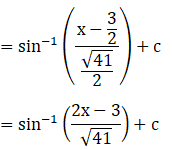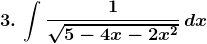Solution: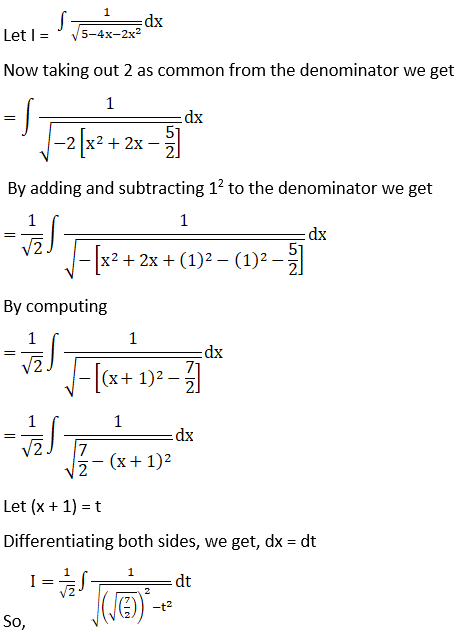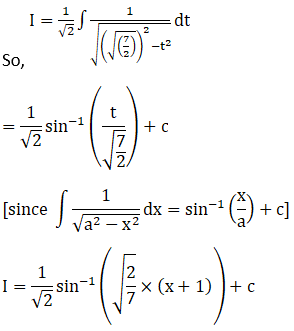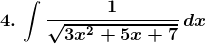Solution: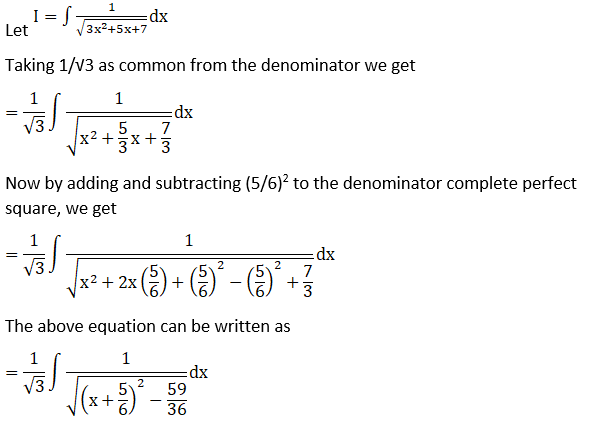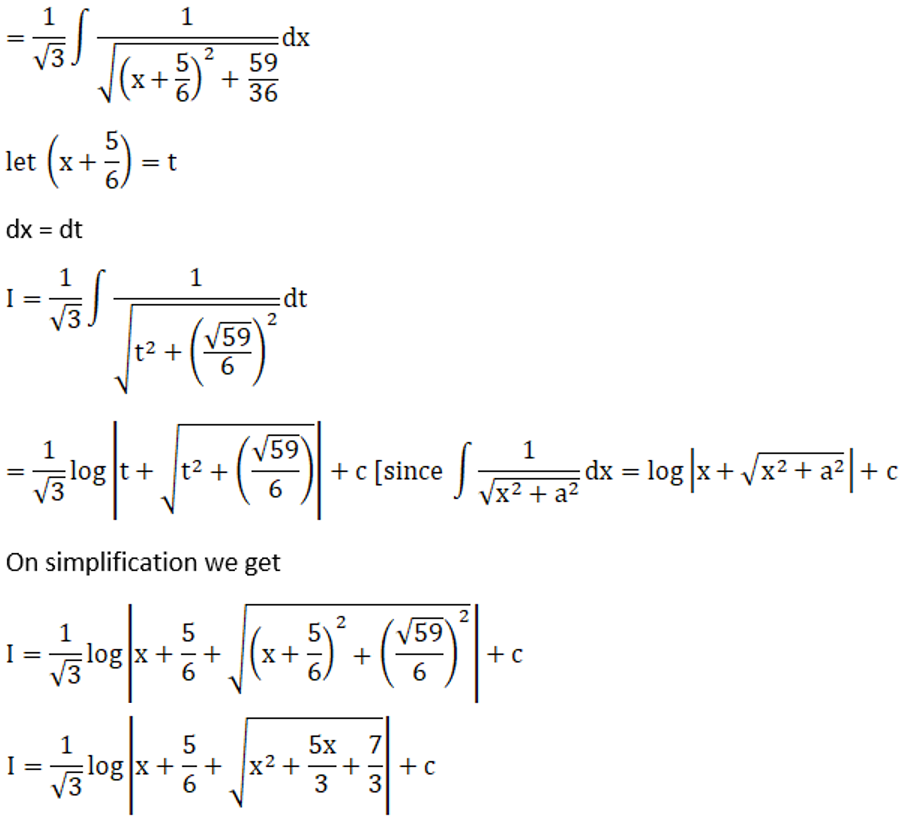### Access other exercises of RD Sharma Solutions For Class 12 Chapter 19 – Indefinite Integrals

Exercise 19.1 Solutions

Exercise 19.2 Solutions

Exercise 19.3 Solutions

Exercise 19.4 Solutions

Exercise 19.5 Solutions

Exercise 19.6 Solutions

Exercise 19.7 Solutions

Exercise 19.8 Solutions

Exercise 19.9 Solutions

Exercise 19.10 Solutions

Exercise 19.11 Solutions

Exercise 19.12 Solutions

Exercise 19.13 Solutions

Exercise 19.14 Solutions

Exercise 19.15 Solutions

Exercise 19.16 Solutions

Exercise 19.18 Solutions

Exercise 19.19 Solutions

Exercise 19.20 Solutions

Exercise 19.21 Solutions

Exercise 19.22 Solutions

Exercise 19.23 Solutions

Exercise 19.24 Solutions

Exercise 19.25 Solutions

Exercise 19.26 Solutions

Exercise 19.27 Solutions

Exercise 19.28 Solutions

Exercise 19.29 Solutions

Exercise 19.30 Solutions

Exercise 19.31 Solutions

Exercise 19.32 Solutions